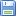Předměty(verze: 941)
Přihlásit přes CASAnglický název: Advanced Statistics Katedra sociologie (23-KS) Fakulta sociálních věd od 2022 letní 8 letní s.:písemná letní s.:1/1, Zk [HT] neomezen / neomezen (15)neomezen ne vyučován angličtina prezenční prezenční http://ICS/ předmět je možno zapsat mimo plánpovolen pro zápis po webu
Garant: PhDr. Ing. Petr Soukup, Ph.D. PhDr. Ing. Petr Soukup, Ph.D. Courses for incoming students
 Soubory Komentář Kdo přidalarticle_sample202006-0001.pdf Article sample PhDr. Ing. Petr Soukup, Ph.D.binary_logreg_HW.doc description of binary logistic HW PhDr. Ing. Petr Soukup, Ph.D.blau_duncan_small.sav Blau Duncan data (SEM practice) PhDr. Ing. Petr Soukup, Ph.D.CFA_WB.sav CFA well-being data (1 factor model) PhDr. Ing. Petr Soukup, Ph.D.flats.omv linear regression for flats - complete computation in JAMOVI PhDr. Ing. Petr Soukup, Ph.D.flats.sav Data file for linear regression PhDr. Ing. Petr Soukup, Ph.D.INET.omv Logistic regression for Internet data PhDr. Ing. Petr Soukup, Ph.D.INET.sav Logistic regression data - Internet usage (WIP 2006) PhDr. Ing. Petr Soukup, Ph.D.INET.xlsx Inet data in MS Excel format PhDr. Ing. Petr Soukup, Ph.D.ISSP_EFA.sav Lecture 8 Exploratory factor analysis - data file PhDr. Ing. Petr Soukup, Ph.D.lecture1_Advanced_2022.ppt Lecture 1 recap PhDr. Ing. Petr Soukup, Ph.D.lecture_11_Advanced_CFA.ppt Lecture 11 CFA PhDr. Ing. Petr Soukup, Ph.D.lecture_12_Advanced_CFA.IIppt.ppt Lecture 11 CFA II PhDr. Ing. Petr Soukup, Ph.D.lecture3_Advanced_2022.ppt Linear regression diagnostics PhDr. Ing. Petr Soukup, Ph.D.LECTURE4_5_Advanced_2022.ppt Lecture 4/5 Logistic regression PhDr. Ing. Petr Soukup, Ph.D.lecture7_LCA_Advanced_2022.ppt Lecture 7 Latent class analysis PhDr. Ing. Petr Soukup, Ph.D.lecture8_LCA_Advanced_2022.ppt Lecture 8 Exploratory factor analysis PhDr. Ing. Petr Soukup, Ph.D.lecure10_Advanced_2022_Path_analysis.ppt Lecture 10 Path analysis PhDr. Ing. Petr Soukup, Ph.D.lecure9_Advanced_2022_Intro_SEM.ppt Lecture 9 Intro to SEM PhDr. Ing. Petr Soukup, Ph.D.library.sav data for multinomial log reg PhDr. Ing. Petr Soukup, Ph.D.logregr_probability_curve.xls How to prepare probability curve in Excel or in Jamovi PhDr. Ing. Petr Soukup, Ph.D.math_4_lca.omv LCA results in JAMOVI PhDr. Ing. Petr Soukup, Ph.D.math_4_lca.sav Lecture 7- data for attitudes for mathematics PhDr. Ing. Petr Soukup, Ph.D.motivation_ind.sav Lecture 7 - Motivation for LCA data PhDr. Ing. Petr Soukup, Ph.D.MOTIVATION_lca.xls Lecture 7 - Motivation for LCA PhDr. Ing. Petr Soukup, Ph.D.Regression_HW.doc description of regression lectures and HW1 PhDr. Ing. Petr Soukup, Ph.D.SEM_reg_cor.sav SEM Intro data PhDr. Ing. Petr Soukup, Ph.D.Titanic.csv Logistic regression data - Titanic PhDr. Ing. Petr Soukup, Ph.D.Titanic.omv Logistic regression for Titanic data PhDr. Ing. Petr Soukup, Ph.D.11_EVS99_CFA.sav CFA EVS data for practising PhDr. Ing. Petr Soukup, Ph.D.11_MI_CESD8.sav MI CESD8 data PhDr. Ing. Petr Soukup, Ph.D.11_MI_intel.sav MI data PhDr. Ing. Petr Soukup, Ph.D.
 Anotace - angličtina
Poslední úprava: PhDr. Ing. Petr Soukup, Ph.D. (06.02.2023)
The course is designed mainly for students of SCS, SOCOME and Sociology study programmes. All other students will be allowed to enter only if the capacity is not full.

This course is an overview course over topics of the use of statistics in sociology, which will cover advanced statistical procedures: linear regression, logistic regression, latent class analysis, exploratory factor analysis and confirmatory factor analysis. All procedures will be demonstrated and practised in Jamovi(2023).
 Podmínky zakončení předmětu - angličtina
Poslední úprava: PhDr. Ing. Petr Soukup, Ph.D. (02.02.2022)

Exam consist of 5 homework, oral exam and draft (article) (every part is evaluated separately 0-100 %). Draft (article) should use classical structure:  problem formulation (derived from theory), hypotheses formulation and statistical analysis for hypotheses evaluation (at least two techniques from sylabus).

Weights for final evalution: every hw 10 %, oral exam 20 % ( for MA students) and draft 30 % (only for MA students).

Final grading: 0-50 % 4 (failed), 51 % - 60 % E, ), 61 % - 70 % D,  71-80 % C, ), 81 % - 90 % B  and 91 % and more A.

 Literatura - angličtina
Poslední úprava: PhDr. Ing. Petr Soukup, Ph.D. (13.02.2023)

Tarling, R. 2009. Statistical Modelling for Social Researchers, Routledge.

 Sylabus - angličtina
Poslední úprava: PhDr. Petr Bednařík, Ph.D. (21.09.2023)

The course will be held in Pekarska building, computer lab 308

Online access is possible via ZOOM:

https://cesnet.zoom.us/j/4677639176?pwd=dVAyWkJISGRrOGp2ZUJRc0I2Y3p2UT09

Meeting ID: 467 763 9176

Passcode: 355749

1.      Introduction to Jamovi. Descriptive statistics and correlation analysis in Jamovi. Missing values, results, handling and replacing. (1 lecture)

2.      Linear regression analysis - simple and multiple regression. Assumptions, model fit, possible modification of regression model. Model evaluation and interpretation. Dummy variables, multicollinearity, influential points, heteroscedasticity. Robust regression.(1st HW)  (2 lectures)

3.      Logistic regression - binary ordinal and polytomous model. Odds, odd ratio, logit. Model evaluation and interpretation. (2nd HW)  (2 lectures)

4.      Latent class analysis (typology from binary and nominal variables). Explanatory and confirmatory approach. Unconditional latent class probability and conditional probability of individual answer. Comparison of models (decision about the number of latent classes). (3rd HW)  (1 lectures)

5.      Exploratory factor analysis. Assumptions, number of factors, Extraction and rotation. Factor weights and interpretation of factors. Factor scores and it’s usage. (4th HW)  (1 lecture)

6. Intro to SEM, correlation, regression and path analysis.

7.      Confirmatory factor analysis for cardinal, ordinal and binary indicators. Model fit indices and criteria. Basic equations and graphical presentation. Modification indices, Bayesian estimation for nominal and ordinal data. (5th HW)  (2 lectures)

Exam consist of 5 homework and oral exam (every part is evaluated separately 0-100 %).

Weights for final evalution: every hw 10 %, oral exam 50 % (for BA students).

Final grading: 0-50 % 4 (failed), 51 % - 60 % E, ), 61 % - 70 % D,  71-80 % C, ), 81 % - 90 % B  and 91 % and more A.